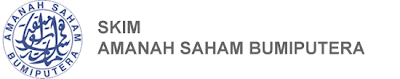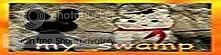## Saturday, December 27, 2008Two of the main components of returns from Amanah Saham Bumiputera (ASB) are the DIVIDENDS and the so called BONUS. Always, Permodalan Nasional Berhad (PNB), will release announcements of the dividend and bonus rates in the media towards the end of the year. Remember that the rates are annual rates. Now, let’s learn on how to ACTUALLY make sense of these rates.

Calculating Dividend

The formula is:
Annual Dividend Rate/12 Months X Average monthly balance for the year

Example:

Say, Dividend rate = 10%, Average monthly balance for the year= RM10,000
So, (10% / 12) X RM10,000 = RM83.33

Take note that the RM83.33 is the monthly dividends. The annual dividend would then be approximately RM999.96.

N.B. = The actual dividends received might differ to a certain degree.

Calculating Bonus

The formula is:
Annual Bonus Rate/12 Months X Average monthly balance for the past 10 years

Example:

Say, Bonus Rate = 1%, Average monthly balance for the past 10 years= RM5,000
So, (1%/12) X RM5,000 = RM4.17

Take note that the RM4.16 is the monthly bonus. The annual bonus for that year should be around RM50.04.

N.B. = The actual bonus received might differ to a certain degree.

Total ASB Returns

The formula is:

Dividends + Bonus

Say, for that year, dividends = RM999.96, bonus= RM50.04
So, total returns = RM999.96 + RM50.04 = RM1,050.00

Calculating Average Monthly Balance

If you were wondering how to calculate the monthly balance, say:

Jan= RM10,000
Feb= RM8,000
Mar= RM12,000
Apr= RM13,000
May= RM7,000
Jun= RM9,000
Jul= RM11,000
Aug= RM10,000
Sep= RM10,000
Oct= RM12,000
Nov= RM8,000
Dec= RM10,000

Add them all up and divide by 12, you’ll get the average monthly balance of RM10,000.Jom tengok Iklan Terbaru Petronas CNY. Klik Sini

#### 1 comment:Anonymous said...

thanks atas pengetahuan tersebut...

## Ini Link saya